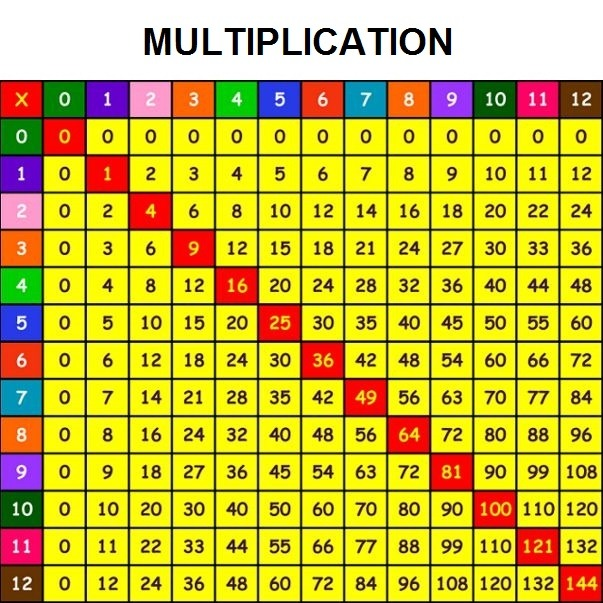# Multiplication table

The multiplication table includes multiplication results for numbers from $1$ to $9$.The multiplication is a lot easier if you know the multiplication table.The result of a multiplication of two numbers is called a product.

The numbers we multiply are called the factors.

The first factor is called the multiplicand and the second is called multiplier.

The multiplication of one-digit numbers is the easiest form of multiplication and must be learned using the multiplication table before moving on to more complex examples.

Now, we are going to look at multiplication of one-digit numbers with two-digit numbers.

Two-digit numbers are composed of numerals  ones and tens.

For example, number $45$ we can write down like:

$\ 45 = 4 \cdot 10 + 5 \cdot 1$

## Multiplication of natural numbers

We are going to start with an example of multiplication of number $8$ with $53$:We started by aligning the multiplier, number $8$, with the number $3$. Then we multiplied each digit of the multiplicand.
We wrote the “ones” digit of each product in the result. If the number has “tens” digit then it will be carried and added to the next product.

The process should look like this:
-> $3 \cdot 8 =24$. Write number $4$ and carry the number  $2$.
-> $5 \cdot 8 = 40$. We adding carried number $2$ to number $40$. The result is number $42$.
-> The final result is number $424$.

Now, we will move on to the multiplication of two-digit numbers.
Let’s take a look at the next example. We will multiply number $71$ with number  $56$:First we multiplied the “ones” digit, number $6$, with number $71$ using the method shown before. The result is number $426$.

Now we multiply number $5$ with  number $71$. The result is number $355$. Note that we shifted the result (355) one digit to the left, and a number $0$ is added at the end. The result is shifted because we multiplied the “tens” digit, number $5$, with $71$.

The process should look like this:
-> $6 \cdot 1= 6$. Write number  $6$.
-> $6 \cdot 7 = 42$. Write number $42$. Now we get number $426$.
-> $5 \cdot 1 = 5$. Write number $5$.
-> $5 \cdot 7 =35$. Write number $35$. Now we got the number $355$, and it is shifted by one digit to the left.

That means that the last digit is $0$.
-> Now we need to add number $426$ to $3550$ and the result is number $3976$.